# Applications of Prime Factorization

Applications of Prime Factorization

Prime factorization is finding the prime numbers that make up the original number.

Let’s take the example of 32. We know that 32 is 8 × 4, but 8 and 4 are not prime numbers. We also know that 8 is 2 × 2 × 2 and 4 is 2 × 2. Since 2 is a prime number, the prime factorization of 32 can be 2 × 2 × 2 × 2 × 2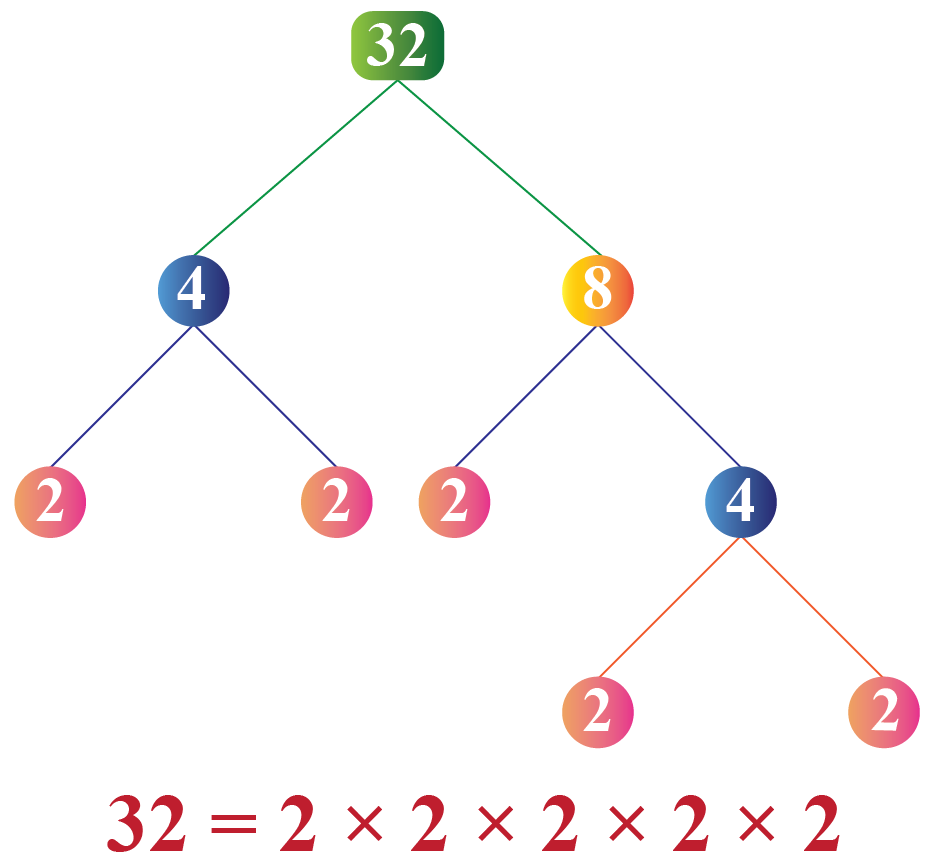Now that you have understood these basics, let us understand prime factorization better.

In this section, we will explore prime factorization as well as the fundamental law of arithmetic using real-life examples.

Check out the interactive simulation to know more about the lesson and try your hand at solving a few interesting practice questions on applications of prime factorization at the end of the page.

## Lesson Plan

 1 What Is Prime Factorization? 2 Thinking out of the Box! 3 Solved Examples on Prime Factorization 4 Challenging Questions on Prime Factorization 5 Interactive Questions on Prime Factorization

## What Is Prime Factorization?

Prime factorization of any number means to represent that number as a product of prime numbers

For example, prime factorization of 36 is the representation of 36 as a product of prime numbers and can be done in the following way-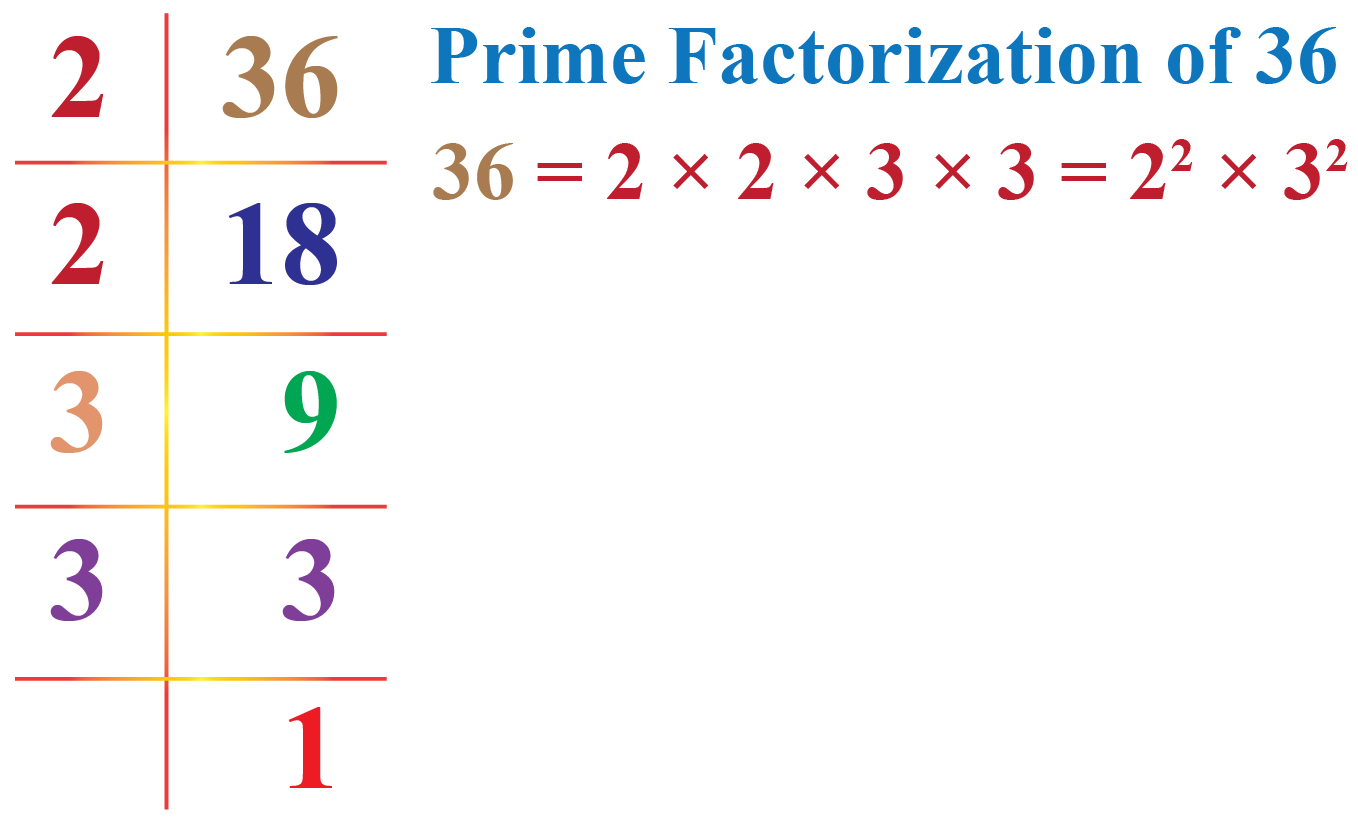Here  2 and 3 are prime numbers.

Let us see the prime factorization of a few more numbers in the following table:

Numbers Prime Factorization
18 $$2 \times 3^2$$
24 $$2^3 \times 3$$
36 $$2^2 \times 3^2$$
60 $$2^2 \times 3 \times 5$$
72 $$2^3 \times 3^2$$
45 $$3^2 \times 5$$
40 $$2^3 \times 5$$
50 $$2 \times 5^2$$
48 $$2^4 \times 3$$
30 $$2 \times 3 \times 5$$
42 $$2 \times 3 \times 7$$

## What Are The Applications of Prime Factorization?

### Cryptography

It is a method of protecting information and communicating it through the use of codes.

Prime factorization plays an important role for coders who want to create a unique code using numbers that is not too heavy for computers to store or process quickly.

### HCF and LCM Using Fundamental Theorem of Arithmetic

To find the HCF and LCM of two numbers, we use the fundamental theorem of arithmetic.

For this, we first find the prime factorization of both the numbers. Next, we consider the following:

• HCF is the product of the smallest power of each common prime factor.
• LCM is the product of the greatest power of each common prime factor.

Example:

What is the HCF of $$850$$ and $$680$$?

Solution:

We first find the prime factorizations of these numbers.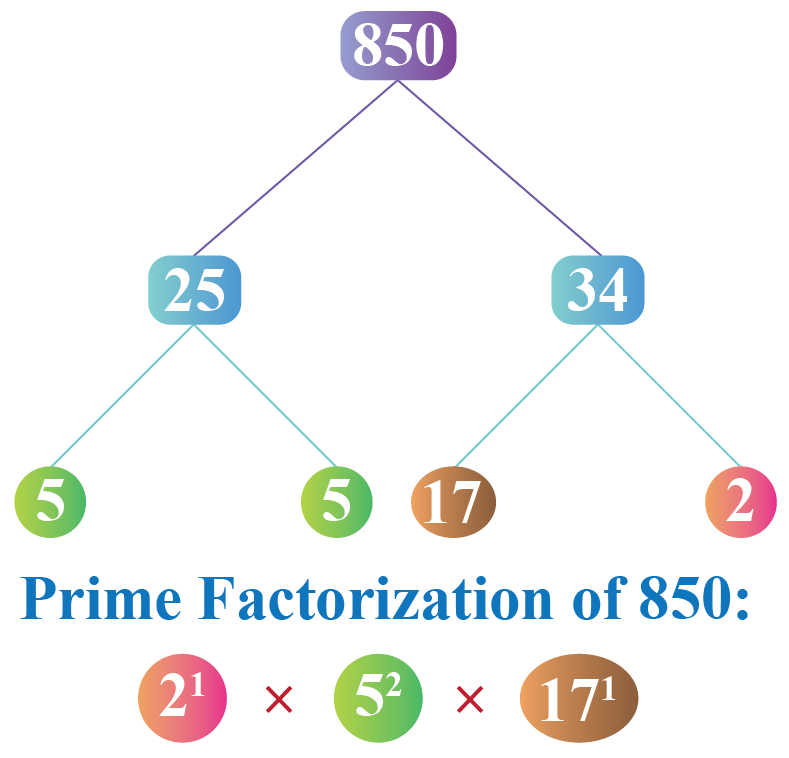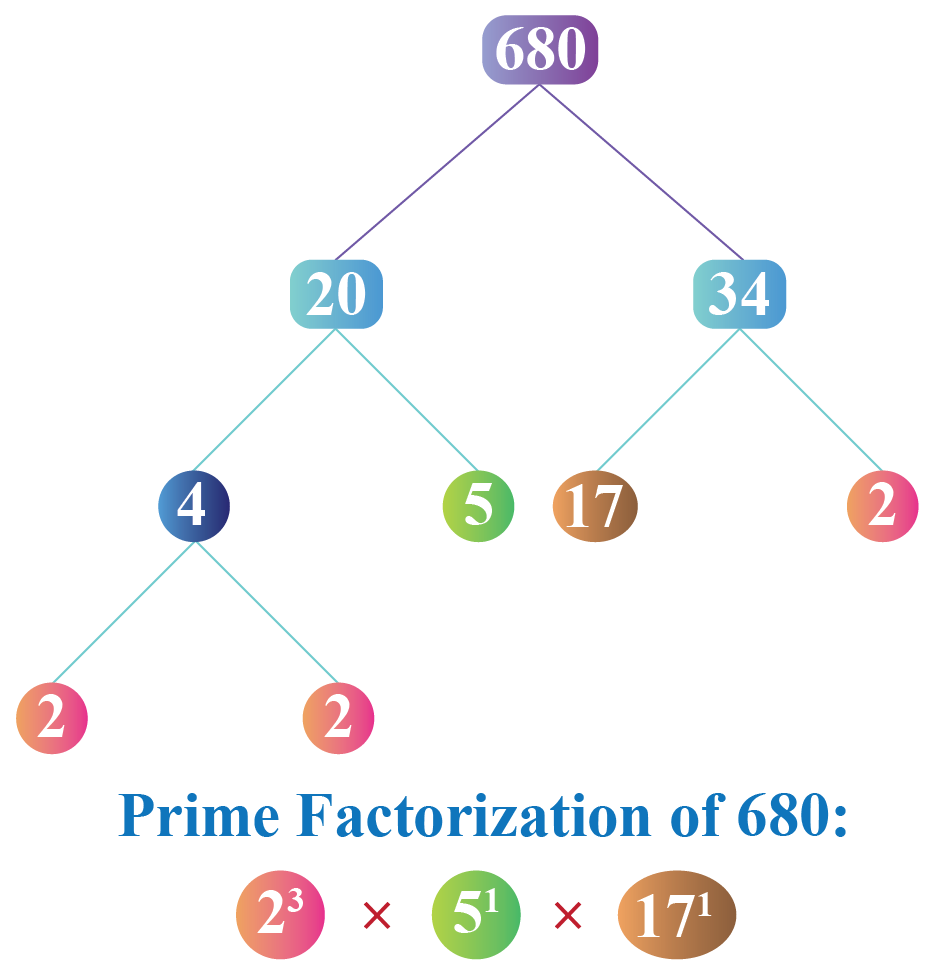Thus:

\begin{align} 850&=2^{1} \times 5^{2} \times 17^{1}\\[0.3cm]680&=2^{3} \times 5^{1} \times 17^{1} \end{align}

HCF is the product of the smallest power of each common prime factor.

Hence,

$\text{HCF }(850, 680) = 2^1 \times 5^1 \times 17^1 = 170$

LCM is the product of the greatest power of each common prime factor.

Hence,

$\text{LCM }(850, 680) = 2^3 \times 5^2 \times 17^1 = 3400$

Thus,

 $\text{HCF }(850, 680) = 170\\[0.3cm]\text{LCM }(850, 680) = 3400$Think Tank
• James has to find the prime factorization of 96 using only the pair factor of 96 which has one odd number, and one even number, in the factor pair.

## Solved Examples

 Example 1

Can you help John to express $$1848$$ as the product of prime factors?  Also, can you tell if this factorization is unique?

Solution

We will find the prime factorization of $$1848$$: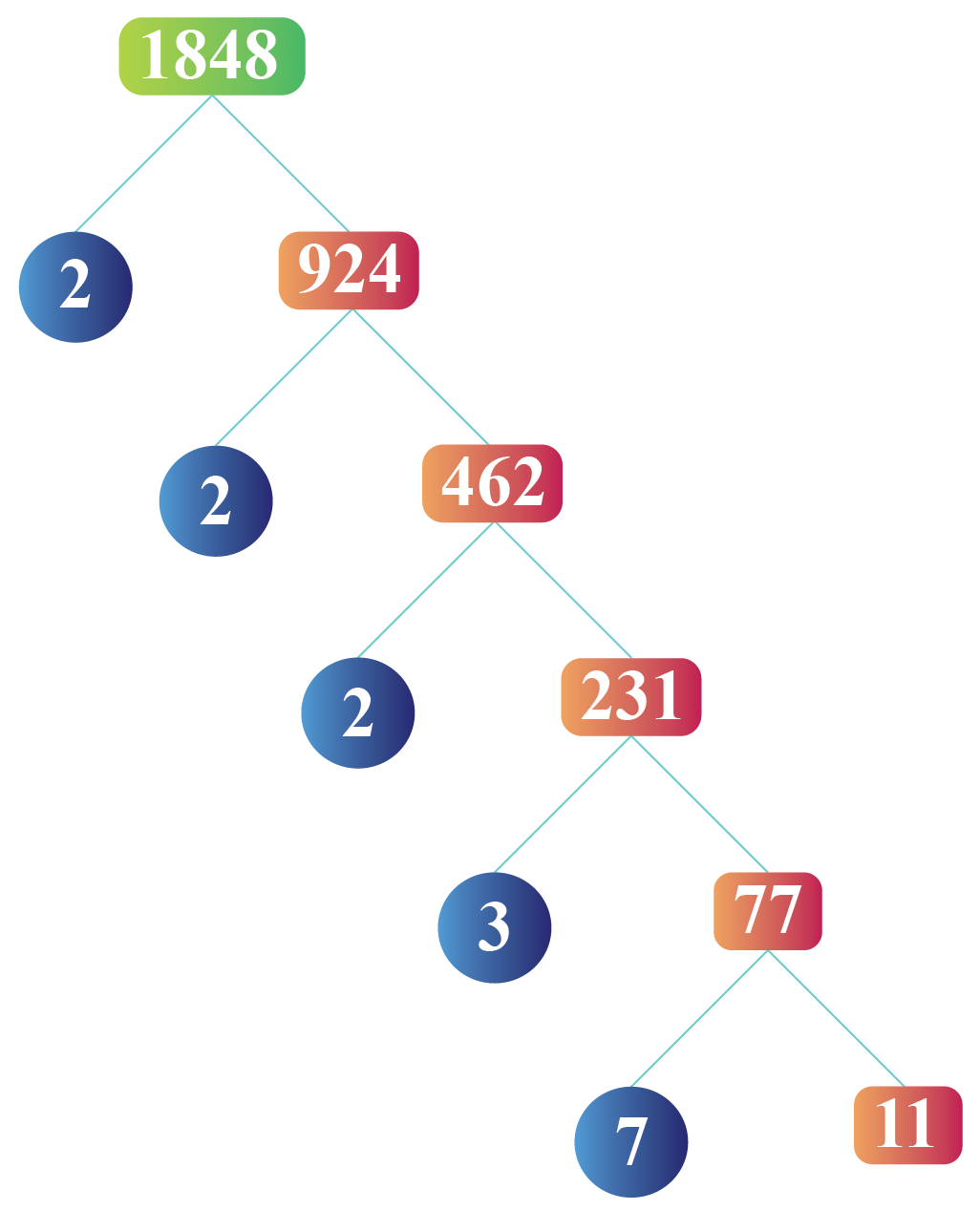Thus,

 $$1848=2^{3} \times 3 \times 7\times 11$$

The above prime factorization is unique by the fundamental theorem of arithmetic.

 Example 2

Sam wants to find the HCF of $$126, 162$$ and $$180$$. Can you help her find the answer to this problem?

Solution

We will find the prime factorizations of $$126, 162$$ and $$180$$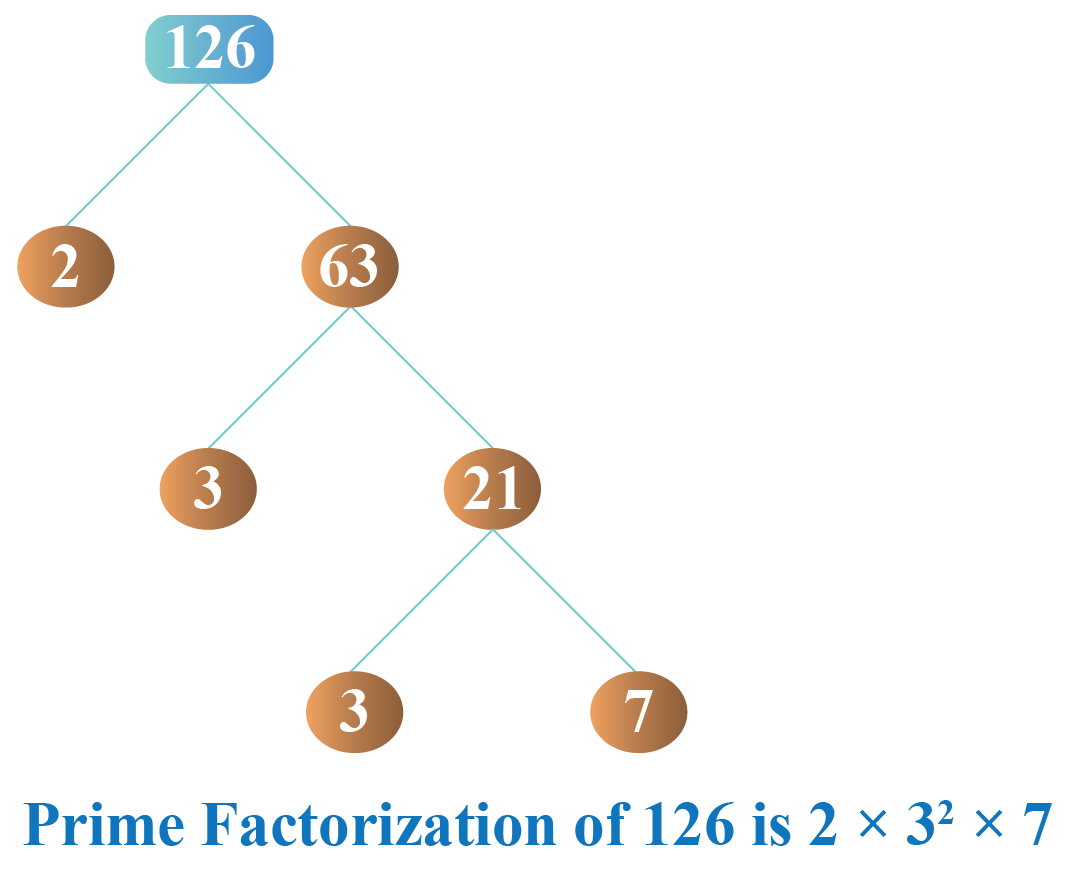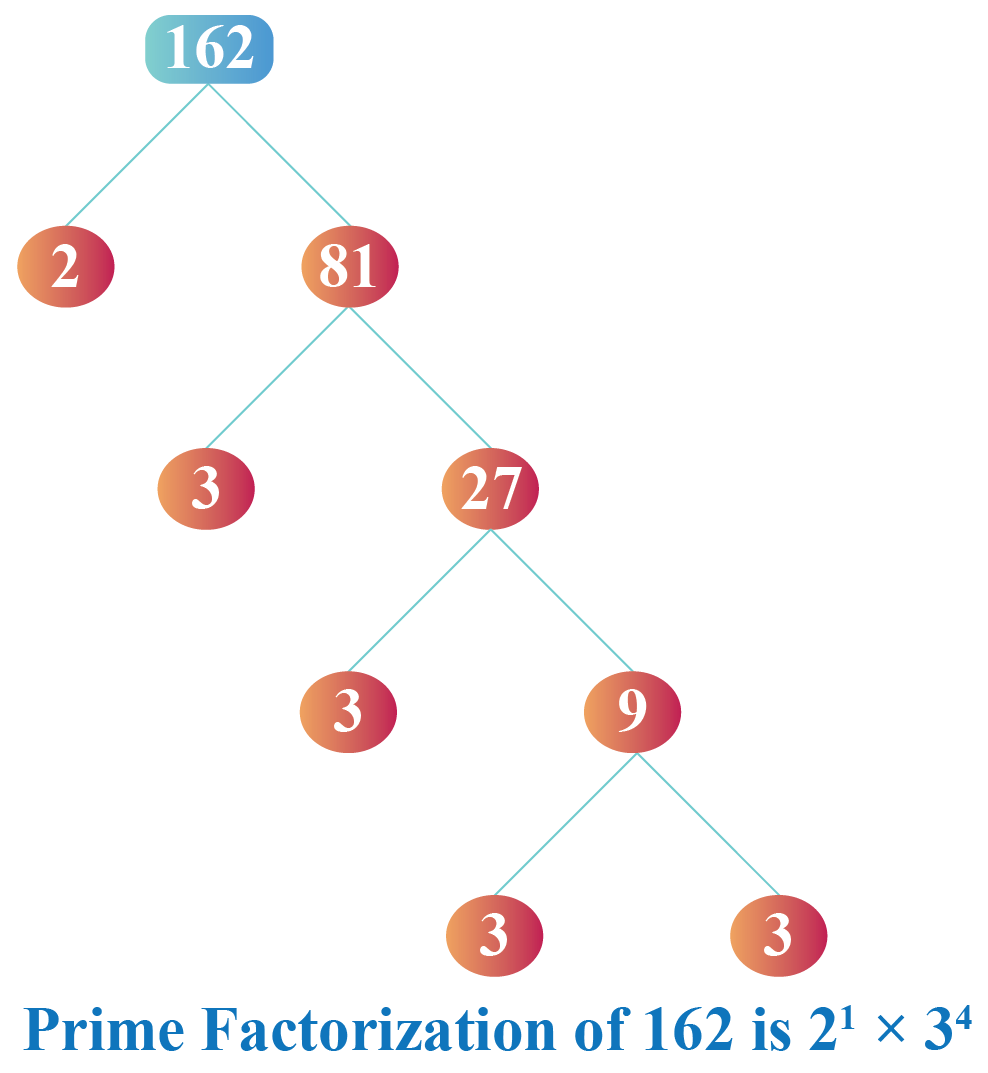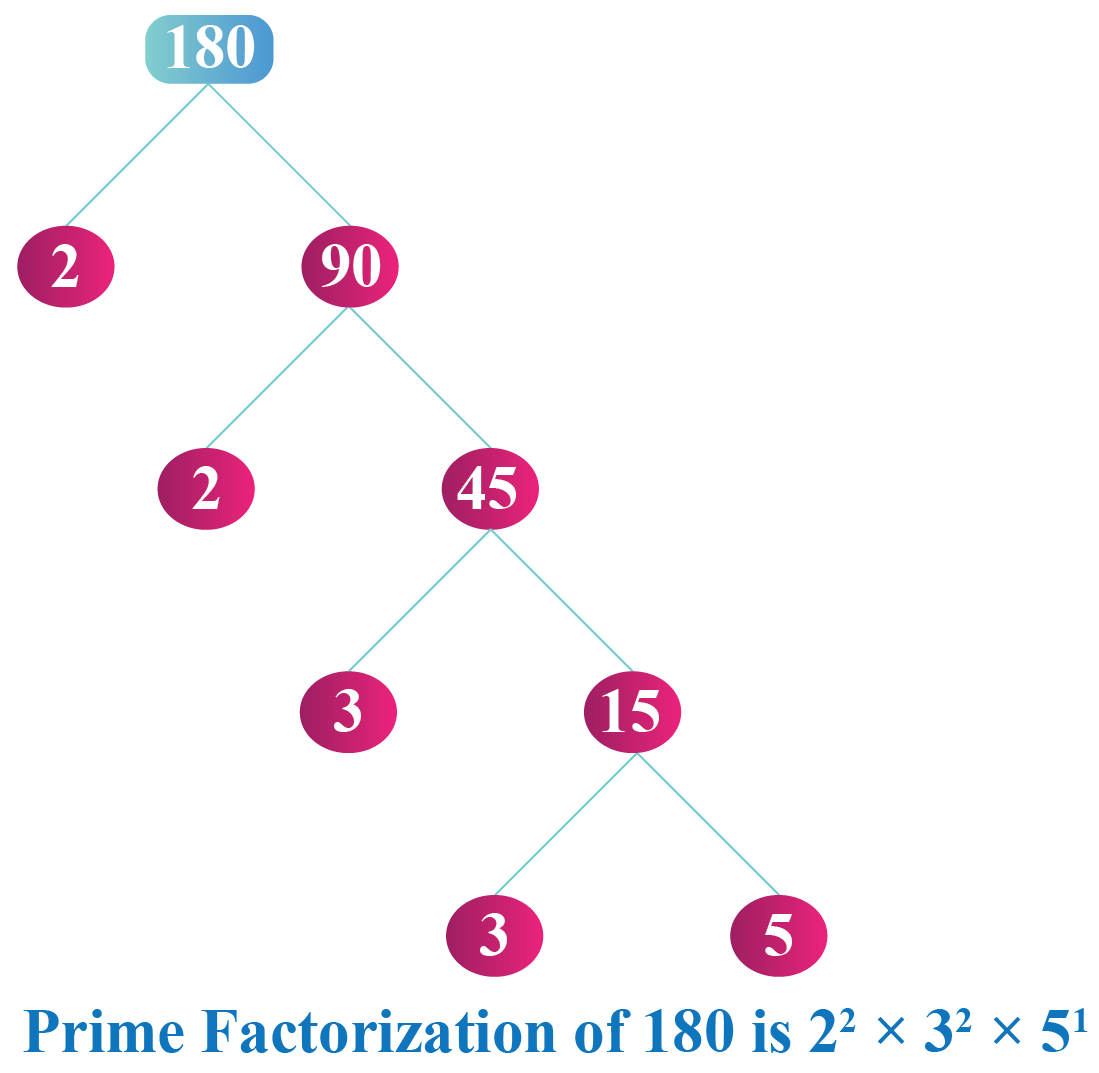Thus,

\begin{align}126&=2^{1} \times 3^{2} \times 7^{1}\\[0.3cm]162&=2^{1} \times 3^{4}\\[0.3cm]180&=2^{2} \times 3^{2} \times 5^{1}\end{align}

The HCF is the product of the smallest power of each common prime factor.

Hence,

$\text{HCF }(126,162,180) = 2^1 \times 3^2 = 18$

 $\text{HCF }(126,162,180) = 18$
 Example 3

Patricia was given a task by her teacher to find the LCM of $$48$$ and $$72$$ using the prime factorization. Can you help her?

Solution

We will find the prime factorizations of $$48$$ and $$72$$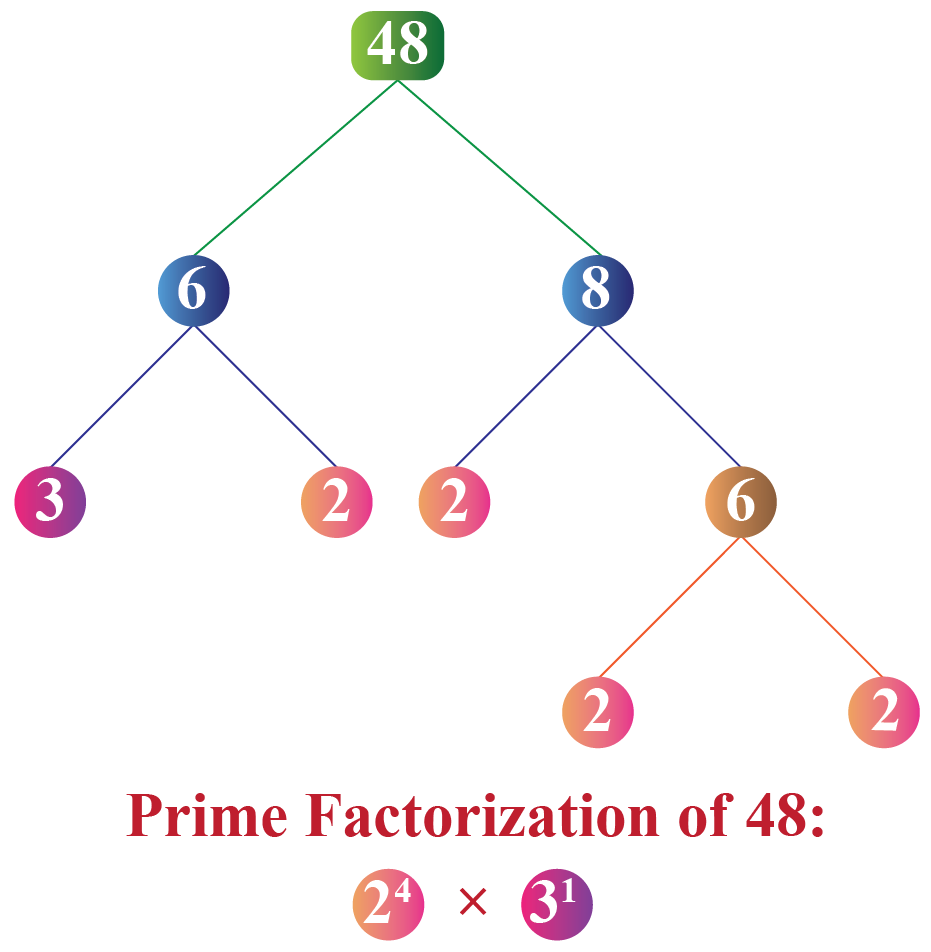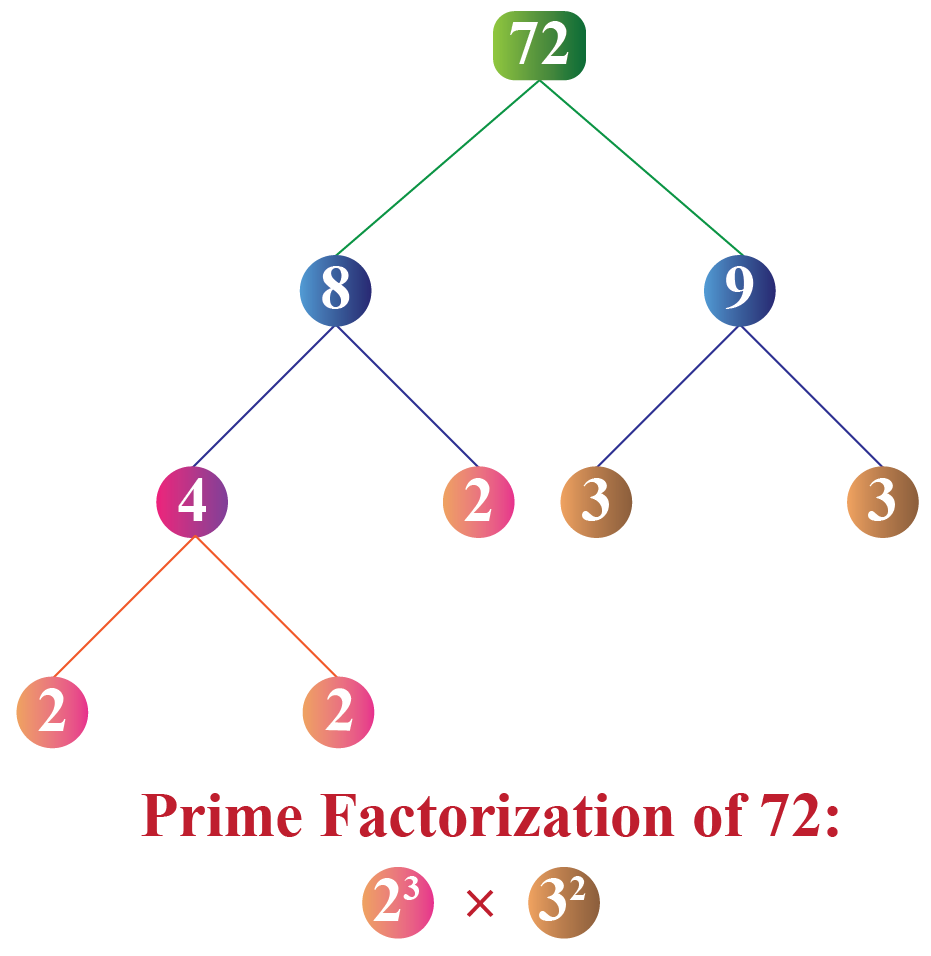The LCM is the product of the greatest power of each common prime factor.

Hence,

$\text{LCM }(48, 72) = 2^4 \times 3^2 = 144$

Thus,

 $\text{LCM }(48, 72) = 144$
 Example 4

Jennifer has to prove that the prime factorization of 40 will always remain the same. Can you help her prove it?Solution

Jennifer can use the division method and factor tree method to prove that the prime factorization of 40 will always remain the same.

Jennifer knows that 40 can be factored in as 5 and 8

8 can further be broken down as a product of 2 and 4

5 is a prime number already.

Hence, she will show the division method and factor tree method in the following way: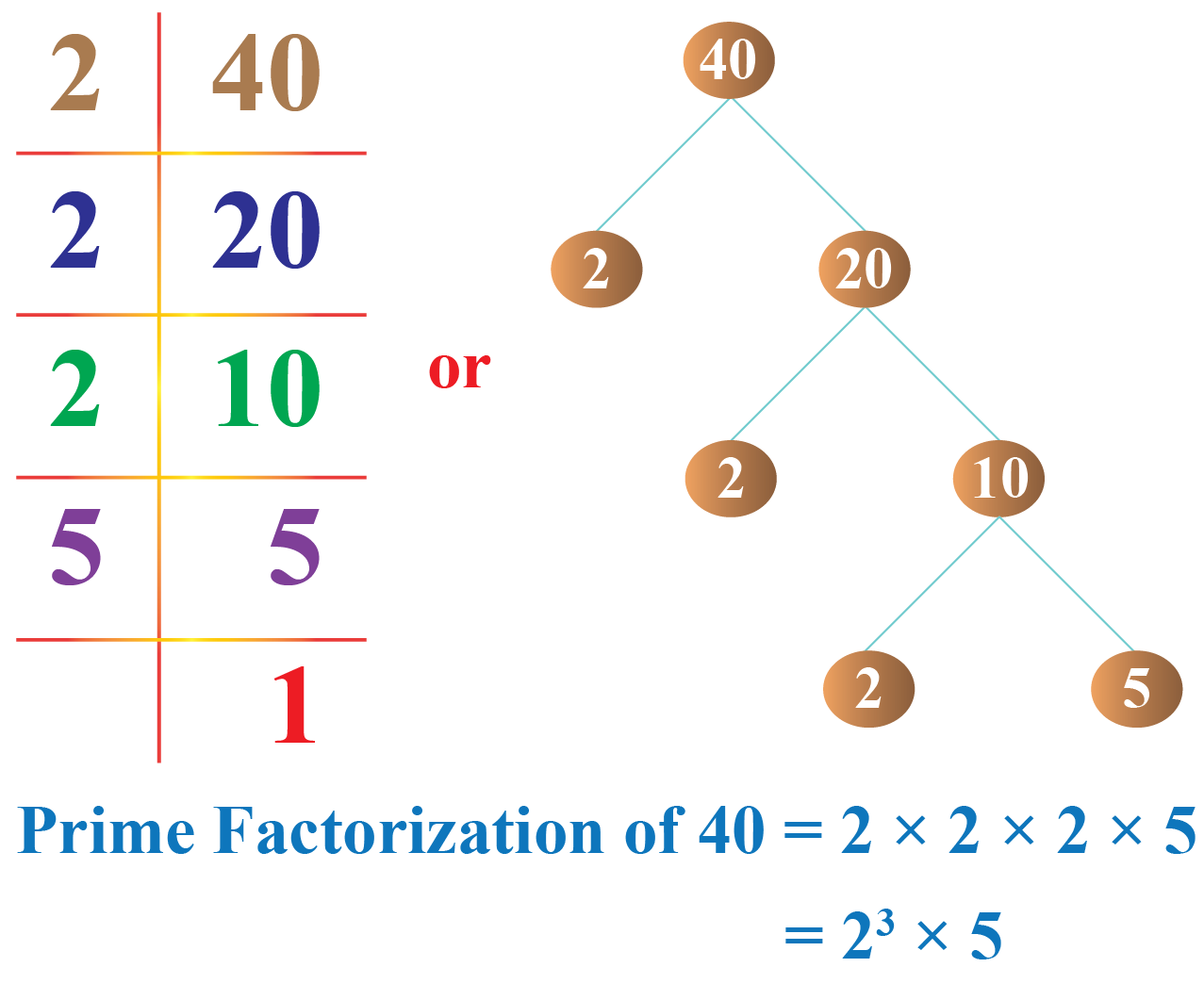$$\therefore$$ This shows that by any method of factorization, the prime factorization remains the same.

 Prime factorization for a number is unique.Challenging Questions
• How would Mary find the prime factorization of 72 using only the pair factors which are co-primes?
• Donald has to split 24 students in his class into different groups with an equal number of students in each. All students cannot be in one group and each group should have more than one student. In how many ways can he form these groups?

## Interactive Questions

Here are a few activities for you to practice. Select/Type your answer and click the "Check Answer" button to see the result.

## Let's Summarize

Mini-lesson targeted the fascinating concept of Applications of Prime Factorization . The math journey around the Applications of Prime Factorization starts with what a student already knows, and goes on to creatively crafting a fresh concept in the young minds. Done in a way that not only it is relatable and easy to grasp, but also will stay with them forever. Here lies the magic with Cuemath.

At Cuemath, our team of math experts is dedicated to making learning fun for our favorite readers, the students!

Through an interactive and engaging learning-teaching-learning approach, the teachers explore all angles of a topic.

Be it worksheets, online classes, doubt sessions, or any other form of relation, it’s the logical thinking and smart learning approach that we, at Cuemath, believe in.

## 1. What are the applications of prime numbers?

The applications of prime numbers are in finding the HCF and LCF of numbers. It is widely used in cryptography as well.

## 2. What is the prime factorization of 24?

Prime factorization of 24 = $$2^3 \times 3$$

## 3. How do you find prime factorization?

Prime factorization of any number can be found out by following two methods:

Method 1: Division method.

Method 2: Factor tree method.

## 4. What is the prime factorization of 13?

Prime factorization of 13 = $$13$$

Arithmetic Integers
grade 10 | Questions Set 2
Arithmetic Integers
Arithmetic Integers
grade 10 | Questions Set 1
Arithmetic Integers
More Important Topics
Numbers
Algebra
Geometry
Measurement
Money
Data
Trigonometry
Calculus
More Important Topics
Numbers
Algebra
Geometry
Measurement
Money
Data
Trigonometry
Calculus
Learn from the best math teachers and top your exams

• Live one on one classroom and doubt clearing
• Practice worksheets in and after class for conceptual clarity
• Personalized curriculum to keep up with school